## Formulas for evaluation of racquet performance

Derivations of these Formulas:

 Torque Impulse Reaction Shock Work Shoulder Pull Impact Force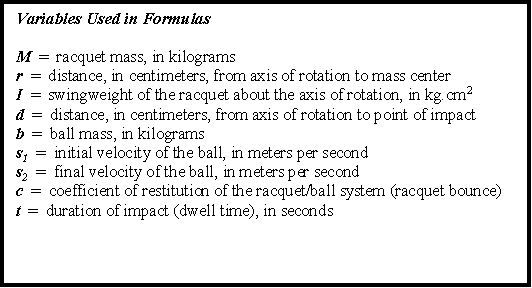Sweet Spot (Center of Percussion) (cm from axis of rotation) = I / (M*r ) Derivation

Moment (Newton.meters about axis of rotation) = M*(r/100)*(9.81) Derivation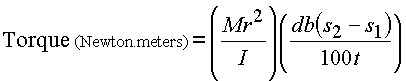Torsion, or Longitudinal Torque = Moment * Torque Derivation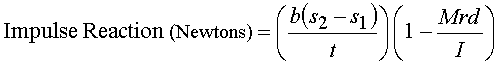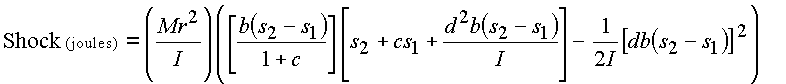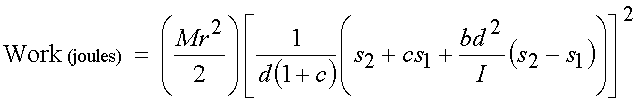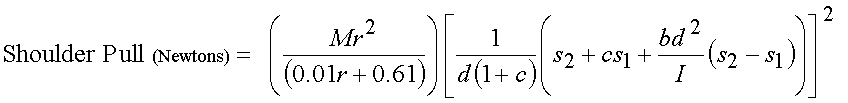Shoulder Crunch (Newtons) = (2/(0.01*r + 0.61))*(Shock) Derivation

Elbow Crunch (Newtons) = (2/(0.01*r + 0.36))*(Shock) Derivation

Wrist Crunch (Newtons) = (2/(0.01*r + 0.08))*(Shock)

Tip Speed (m/s) = ((2/M) * (Work) * (e / r)²)^½ e = distance from axis to tip, in cm Derivation

Impact Force (Newtons acting at mass center due to impact) = (M / t)*{(1 + c)*[(2/M)*(Work)]½ - (r/d)*(cs1 + s2)} Derivation

Parallel Axis Theorem for converting RDC swingweight (I10) to swingweight about the axis specified for the benchmark condition (I7 or I5, or Ia which is the I used in the foregoing formulas):

I = Ia = I10 + M*[2*(10 - a)*r - (10 - a)²] Derivation

New Balance Point distance (x) from original balance point, resulting from adding weight (m) at a distance (y) from the original balance point of a racquet having an original weight (M).

x = y * (m / (M + m)) Derivation